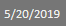I was working on a customer’s data where I wanted to get the output from a table, but have it stored as a value or item.

I am sure that in the comments there might be a possible way to do this more efficiently, but below is how I solved this challenge.

As always it is best to work through with an example.

• I want to get the Max date from my data
• Then use this to build a period table based off this date.
• NOTE: I know that I could possibly use the current date, but in my situation if the data did not get refreshed all the reports would be wrong, because the max date would no longer be correct.
• This was the output I was looking for below, this would allow me to use it in my Period Table.
•• As you can see above it is the value or item, but it is not a table or list which is what I was looking for.

## How I solved my challenge

Before getting this working, I tried to convert the output of my table to a list, or table or transpose or anything to get it to display the way I wanted.

This was my table loaded with the last date for data.I used the following code below.

```let
Source = Table.FromRows(Json.Document(Binary.Decompress(Binary.FromText("i45WMjIwtNQ1MNU1MlCKjQUA", BinaryEncoding.Base64), Compression.Deflate)), let _t = ((type text) meta [Serialized.Text = true]) in type table [LastDataDate = _t]),
#"Changed Type" = Table.TransformColumnTypes(Source,{{"LastDataDate", type date}}),
in
MaxDate
```

The only line I need to refer to is line 4

• I referenced the previous line and then got the first value {0} from my column shown above which was called [LastDataDate]

This then allowed me to get the output I required.Which then meant that I could solve my challenge and use the above value or item in another table as a referenceUpdate: 30 May 2019

I got a comment from Chris at precisiondax.com where he had an alternative solution which has the same result below.

```let
Source = Sales
,MaxDate = Date.From( List.Max(Source[Date Column]) )
in
MaxDate```

## Conclusion

I had a challenge and by using Power Query I found a way to get the output I required.

This once again shows how powerful Power Query is and how it can shape any data you require.

As always please leave any comments or suggestions if there is a better way to get this done, and I will update my blog post.

You can find the above workbook here: Output from a table as a value or an item.pbix